# Topic 12 Simple linear regression

## 12.1 Motivation

Recall, from last time, that our goal is to address research questions:

• Does college degree influences future income?
• Does frequent exercise influence life expectancy?
• Does eating healthy influences the probability of getting sick?
• Does attending labs influence my chances of doing well on the exams?

Even though it is not simple to make causal claims, a good first step is to explore simple correlations between variables, this is what we did last week.

A second step is to see how well our independent variable predicts our dependent variable. This is the purpose of simple linear regression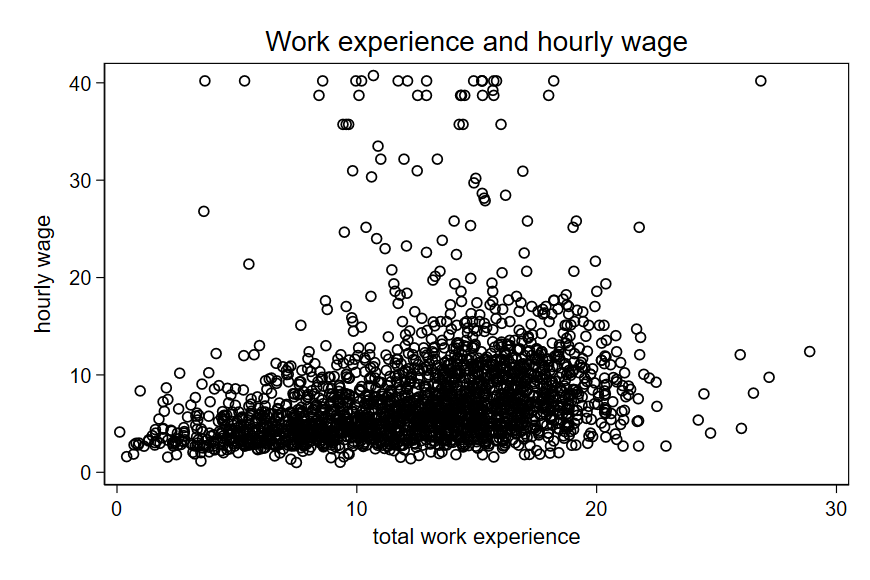## 12.2 Intuition

Linear regression is a systematic way to draw a line that best fit the observations we have.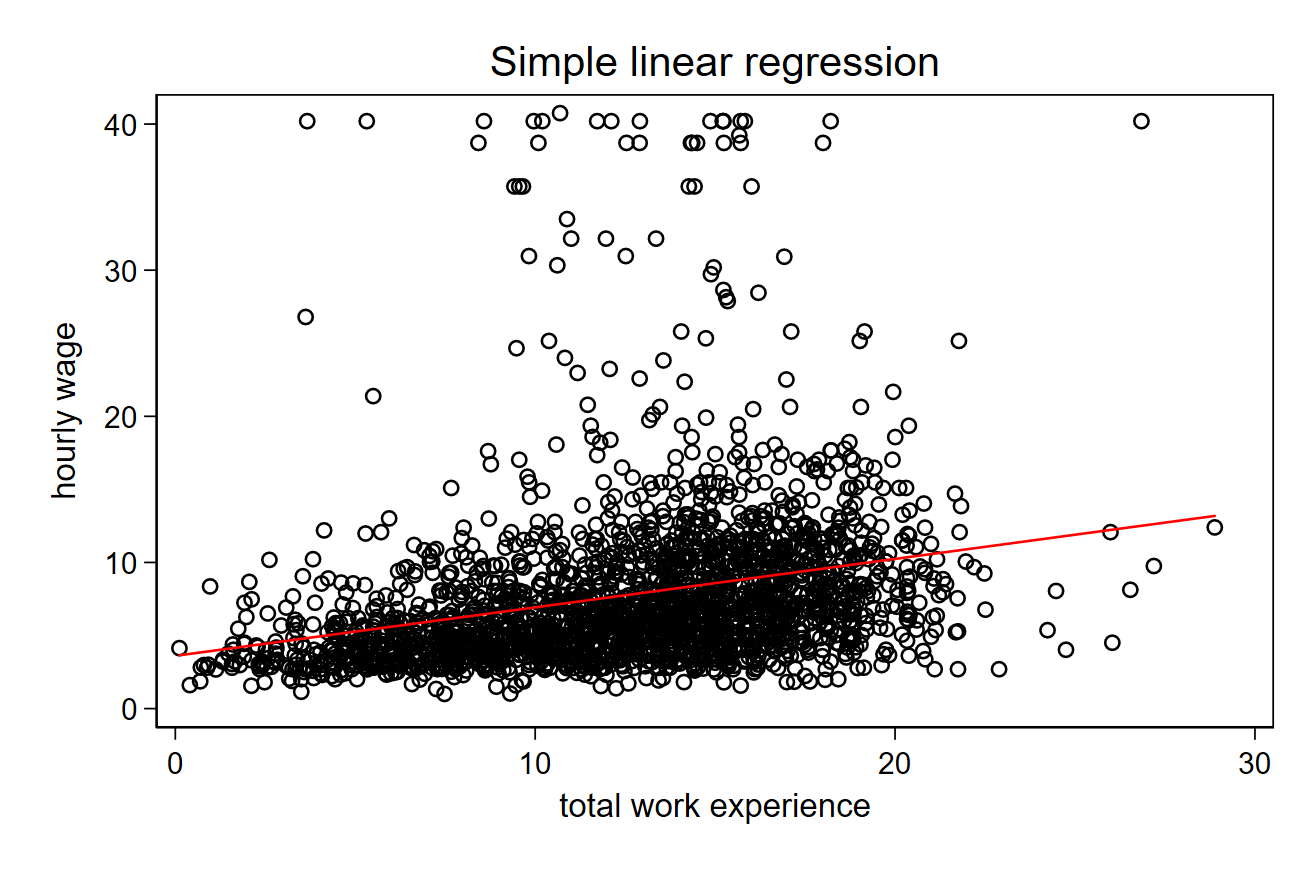Have you ever learned about the equation of a line? (this is simple linear algebra):

$y = a \cdot x + b$

See here for an example.

In summary:

• $$a$$ is the slope of the line.
• $$b$$ is the intercept.

When we perform a regression, we calculate the equation of a line. $$y$$ is our dependent variable and $$x$$ is our independent variable.

$y = \beta \cdot x + \epsilon$

Important:

• Regression is good for prediction, because we can plug our independent variable (x) and see what value we predict for our dependent variable (y).
• Whatever is not well predicted by our regression coefficient ($$\beta$$) is captured by the residual ($$\epsilon$$).

## 12.3 Minimizing sum of square residuals

If you are curious, this is how it works: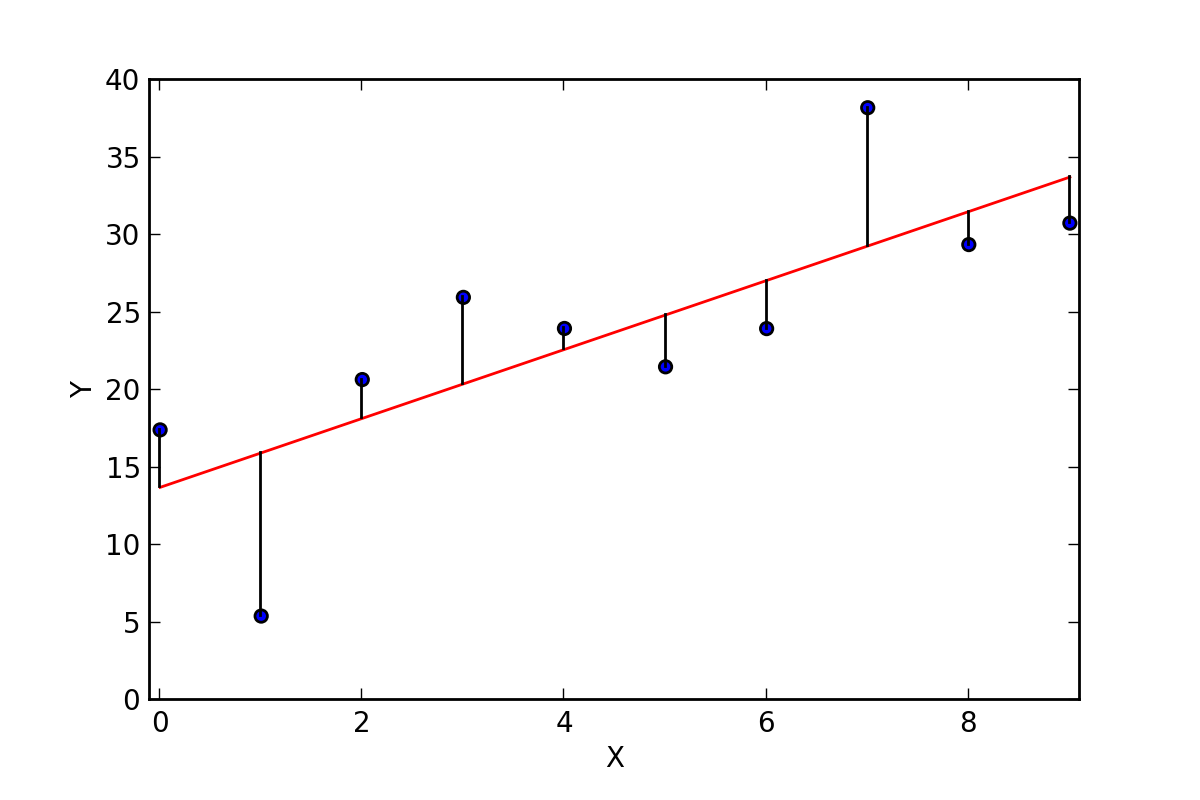### 12.3.1 Formula and calculations

For your homework, this is what you should do:

$\hat{\beta} = \frac{ \sum_{i=1}^n (x_i - \bar{x})(y_i - \bar{y}) }{ \sum_{i=1}^n (x_i - \bar{x})^2}$

$$x$$ $$y$$ $$(x - \bar{x})$$ $$(x - \bar{x})^2$$ $$(y - \bar{y})$$ $$(x - \bar{x})(y - \bar{y})$$
$$\bar{x}$$ $$\bar{y}$$ Sum = Sum =

Use the table above to calculate the b coefficient.

Then plug any two values (x,y) and calculate your intercept (a):

$y = b \cdot x + a$

$y - b \cdot x = a$

## 12.4 Interpretation

• The calculated coefficient tells how many units your dependent variable change if you change your independent variable by 1 unit.
• You should look at your p-value to see the statistical significance of your coefficient. This is calculated by a simple t test.
• Null hypothesis: regression coefficient = 0.
• Your R-squared tells you the percentage of variation in $$y$$ that your $$x$$ explains.

## 12.5 Problems with linear regression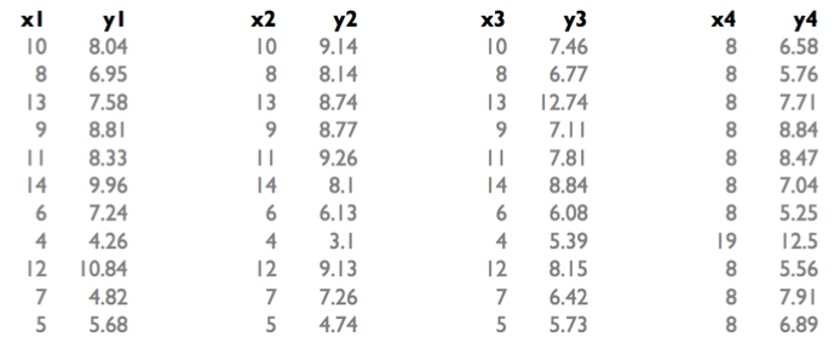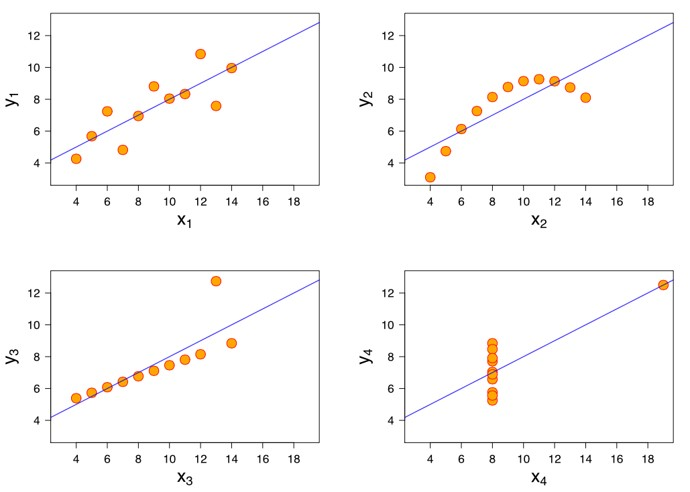This is called the Anscombe’s quartet.

We also have the problem of confounding variables we discussed last time. But this is beyond this course (multiple linear regression).

## 12.6 Exercise

Using the school dataset, I will illustrate a regression of “api 2000”, a measure of academic achievement, on “avg class size 4-6”.

In other words, we have the following equation:

$api00 = \beta \cdot acs_46 + \epsilon$

Also using the dataset “school_data.sav”, perform and interpret the following simple linear regressions:

• $$api00 = \beta \cdot grad_sch + \epsilon$$
• $$api00 = \beta \cdot enroll + \epsilon$$